# Correlation and R-Squared

What is R2? In the context of predictive models (usually linear regression), where y is the true outcome, and f is the model’s prediction, the definition that I see most often is: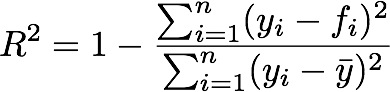In words, R2 is a measure of how much of the variance in y is explained by the model, f.

Under “general conditions”, as Wikipedia says, R2 is also the square of the correlation (correlation written as a “p” or “rho”) between the actual and predicted outcomes:I prefer the “squared correlation” definition, as it gets more directly at what is usually my primary concern: prediction. If R2 is close to one, then the model’s predictions mirror true outcome, tightly. If R2 is low, then either the model does not mirror true outcome, or it only mirrors it loosely: a “cloud” that — hopefully — is oriented in the right direction. Of course, looking at the graph always helps: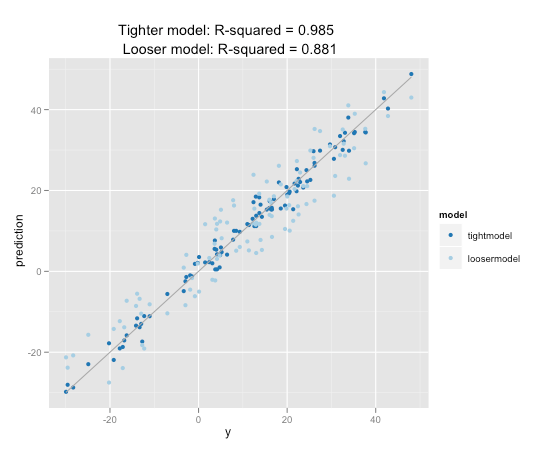The question we will address here is : how do you get from R2 to correlation?

If you look at the two equations for correlation and R2, you can see that the relationship between them does not hold for general f and y. In particular, correlation is far more invariant to scaling. For correlation, all of the following relations are true:But only the last relation is true for R2. So in general, the two cannot be functions of each other.

However, we are making a specific assumption about f: it is the output of a predictive model. In fact, we are actually making several specific assumptions;

1. f is the model that minimizes squared-error loss
2. Because it is the optimum (in the sense of item 1), there is no shift of f that will improve the fit.
3. Because it is the optimum (in the sense of item 1), there is no scaling of f that will improve the fit.

We can express the above assumptions as follows: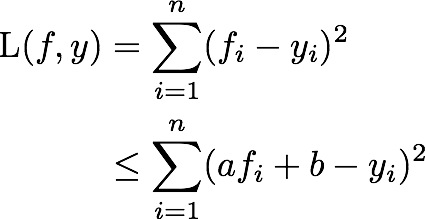If we express the last line as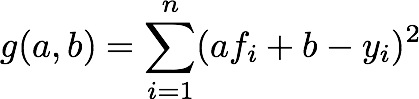Then loss is optimized at g(1,0).

Since g(1,0) is the optimum, then the derivatives of g are zero here:From the partial with respect to a, we get that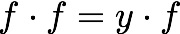and from the partial with respect to b, we get that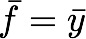(since the mean is just the normalized sum).

Now, let’s shift the coordinate system so that y (and f) are equal to zero. This makes the equations much simpler, and doesn’t affect the generality of the result.

The equation for R2 is nowAnd the equation for correlation is nowAnd we are done.

Notice that this result is true for any model fit that meets the assumptions that we outlined above (squared-error loss, optimality under shifting and scaling). Linear regression (with an intercept) fits this criterion, but so can other model-fitting techniques — generalized additive models, polynomial fits, decision (regression) trees, ensemble methods — if the proper loss function is used.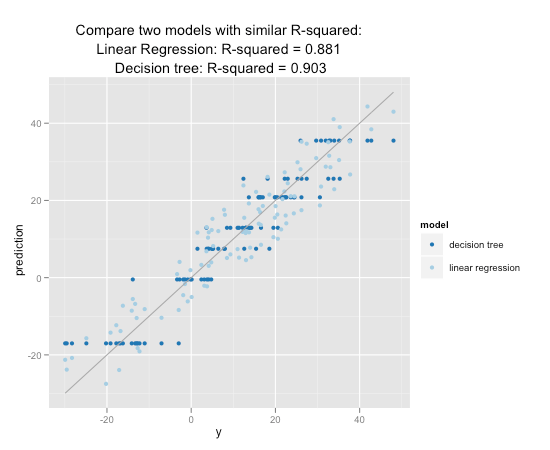To repeat: for optimal models (under squared-error loss, shift and scale invariance), R2 is the square of the correlation between the true and predicted outcomes. This relationship is not true for general f and y.### Nina Zumel

Data scientist with Win Vector LLC. I also dance, read ghost stories and folklore, and sometimes blog about it all.

1.Ritesh says: